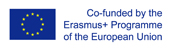This project has been funded with support from the European Commission.
This web site reflects the views only of the author, and the Commission cannot be held responsible for any use which may be made of the information contained therein.

Select language   >   IT EN RO PL LT

### Probability

Subject taught
- Math

Type of Product
- Exercises
- Online module
- Slides

Language Skills Developed
- Listening
- Writing

Transferable/Scientific Skills Developed
Student will develop self-motivation, analytical skills, problem-solving skills

Description
General aims:
Student will be able to determine the probability of an event using the results of an experiment. Student will be able to solve basic mathematical problems connected with probability which can be used in the real life. Student will know the language and patterns associated with probability. Student will develop positive learning habits such as organisational and problem-solving skills.

Linguistic aims:
- to read English with ease
- to understanding content
- to learn from written texts
- to master tools for gathering information
- to express understanding and experiences
- to enable students to understand topic correctly
- to translate words connected with probability in English into the mother tongue using one’s own words.

Subject specific aims:
- to familiarise students with the ways in which we talk about uncertainty
- to engage students in activities which will give them contact with the ideas of probability
- student is able to determine the probability of an event using the results of an experiment
- student is able to determine probabilities using matching outcomes/total outcomes
- student is able to understand the concept of a 'sample space'
- student is able to identify the sample space for both a single event and two combined events (e.g. adding two dice) and use to calculate probabilities
- student is able to understand the difference between experimental and theoretical probabilities
- student is able to calculate experimental probabilities/relative frequencies and estimate the most likely count given the number of trials and a probability .

Target group age:
Students from primary school (14-15 years old), before using the resource they need to know how to do basic operation with fractions, decimals and percentages. What is more, these slides can be used by anyone who wants to do extend one’s knowledge about probability . Also, by grade teachers who wants to teach probability using English.

Level of competence in English (CEFR)
B1/B1+

Time required to use the resource with the students:
The teacher can use the resource with the students for 3-5 lessons. There are
40 slides.

How to use it
Before using the video during one’s classes, the students should be able to do basic operation with fractions, decimals and percentages.

The teacher highlights some new words for students in English and students should find the translation these words into the mother tongue and write down the new words in English in the notebook with the right translation in their own words.
Teacher can use slides during one’s lesson as a lecture but it can be also a homework for a student to practice a material at home. There is theory and practice, students can test their understanding and do revision.
The teacher presents the slides and does pauses in certain moments to give students opportunity to make notes, asks questions and develop some presented problems. The teacher should give them a feedback and show students more examples on the black table. Students should do similar exercises in pairs. The teacher gives a feedback to the students and helps them.
Possible difficulties for the students
Students who have poor knowledge of English can have problems with understanding the content. Students who has problems doing basic operations with fractions and decimals can face difficulties in solving math problems with probability.